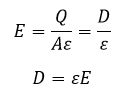# What is Electric Field, Electric Field Intensity, Electric Field Density

## What is Electric Field

Electrical Field E is defined as surrounding a charge particle where it can experience a force by another charge particle, the force may be repelling or attracting each other. Or electric field defined as the space around the charge particle which experience a force by another charge particle.

Or In Simple Words:

As per Coulomb’s law When a charge particle enters into another charge particle’s the electric field then it experiences a force. In other words, the electric field is the region around a charge particle where the lines of force can be felt by another charge by getting repulsed or attracted as per their sign of charge

Repulsive Force:

Let us consider a unit positive charge +q a test charge is placed near a positive charge +q, the unit positive charge will experience a repulsive force, one charge moves away from the other charge. Which means if you bring test charge near to another positive charge +q then the line of force is directed outward. please refer the drawing. Here the line of force on the test charge is represented by the imaginary line of force and the force lines are starting from +ve charge and ending with –ve charge. Also here you another one important thing is the lines of force come out from positive charge. And in a negative charge, the lines of force come into this charge.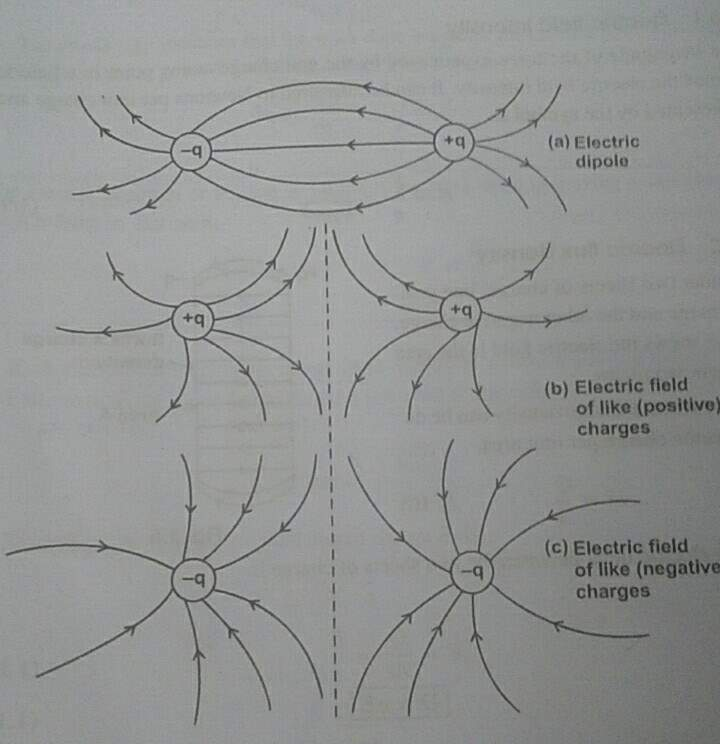+ve Charge => the line of force come out from it

-ve charge => the line of force come into it

Suppose if you take both as –ve charge -q means you will get the repulsive force and the line force is directed inward. You check the line force direction in the drawing.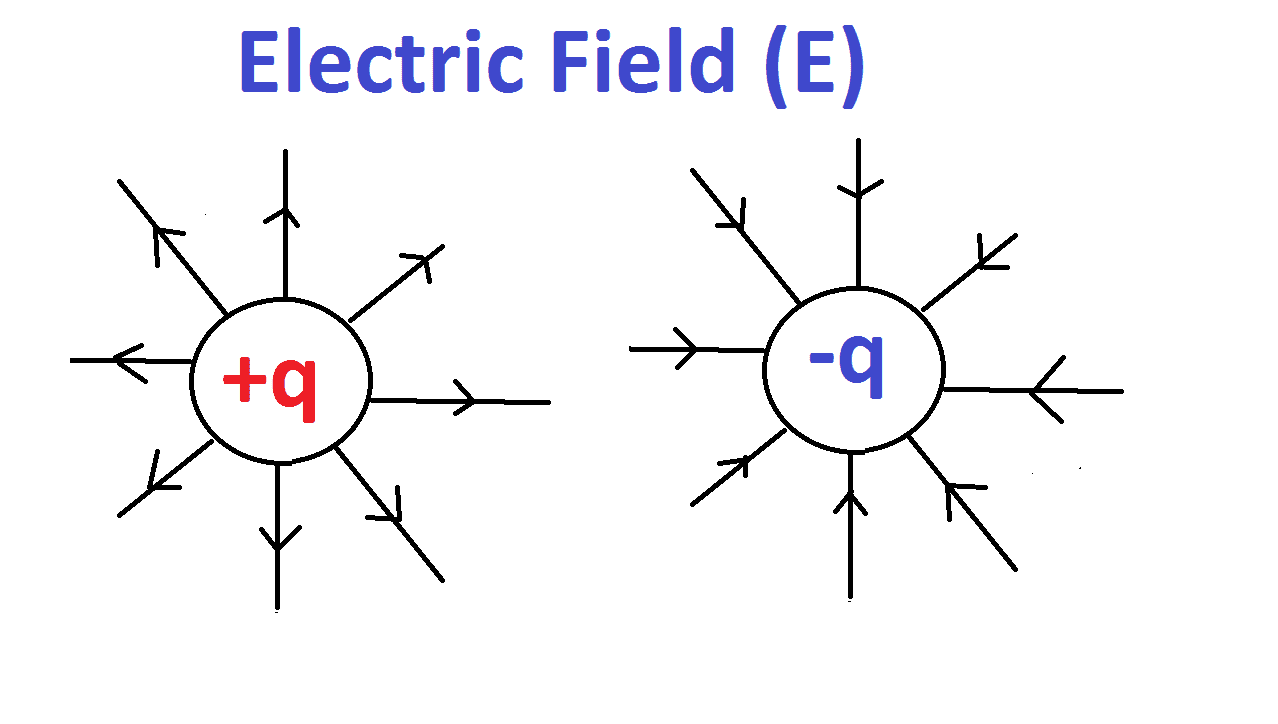### Electrical dipole: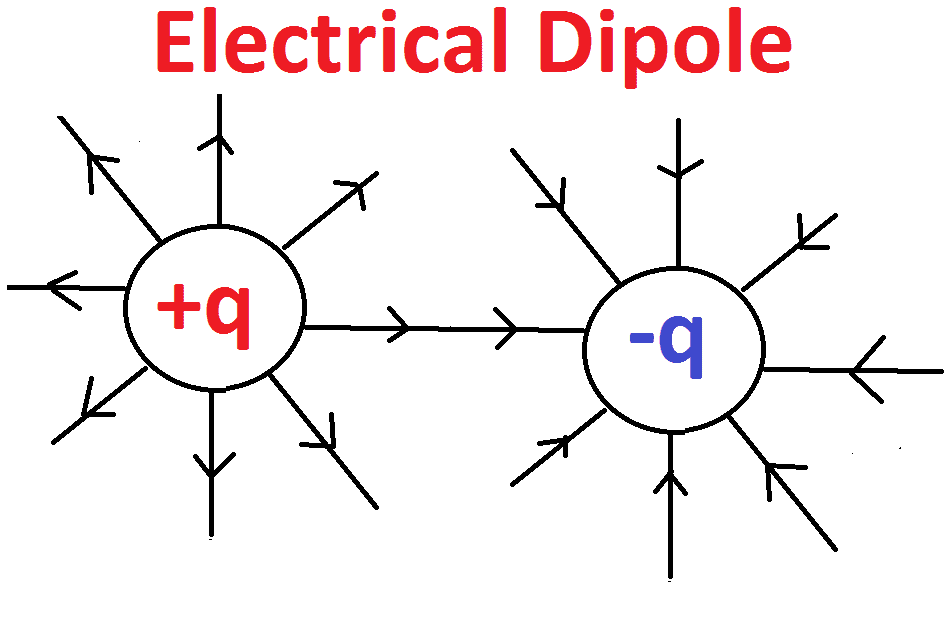Here you consider two charges which should be equal in magnitude but opposite sign (positive and negative) at a fixed distance, here the line of force is starting from +ve charge particle and end with –ve charge particle. During this action, you will get the attractive force. This is called electrical dipole.

Let’s calculate the mathematical expression for Electric field (E):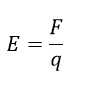Let us consider a test charge particle +q at a point. By the definition of the electric field, the force around the charge at a point is called electric field. This electric field has both magnitude and direction. Here the direction of electrical field E is defined as the direction of the force exerted by a +ve test charge. The magnitude of electric field E is calculated by the ratio between force acting on the test charge and the charge itself.

These Lines are also called as electric field line, it has some unique property. Let see

## Properties of Electric Lines of Force or Field Lines:

1) The Force Lines are only imaginary part, practically we cannot see them.

2) A unit positive charge placed in the electric field tends to follow a path along the field line if it is free to do so.

3) Electric field lines starts from positive charge and end on a negative charge, so they do not form closed curves. These electric field lines do not start

4) The tangent to an electric field line at any point gives the direction of the electric field at that point.

5) These electric field lines never intersect each other.

6) Electric lines of force are closer (crowded) where the electric field is stronger and the lines spread out where the electric field is weaker.

7) Electric lines of force are perpendicular to the surface of a positively or negatively charged body.

8) Electric lines of force contract lengthwise to represent attraction between two, unlike charges.

9) Electric lines of force exert lateral (sideways) pressure to represent repulsion between two like charges.

10) The number of lines per unit cross-sectional area perpendicular to the field lines (i.e. density of lines of force) is directly proportional to the magnitude of the intensity of electric field in that region.

11) Electric lines of force do not pass through a conductor. Electric field lines can pass through an insulator.

## Electric field intensity:

As we said in the above equation the magnitude of the force experienced by the unit charge at a point in a field is called as electric field intensity.

Newton (N) per C (Coulomb) is the SI unit for electrical field intensity (E).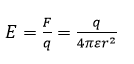## Electric Field Density: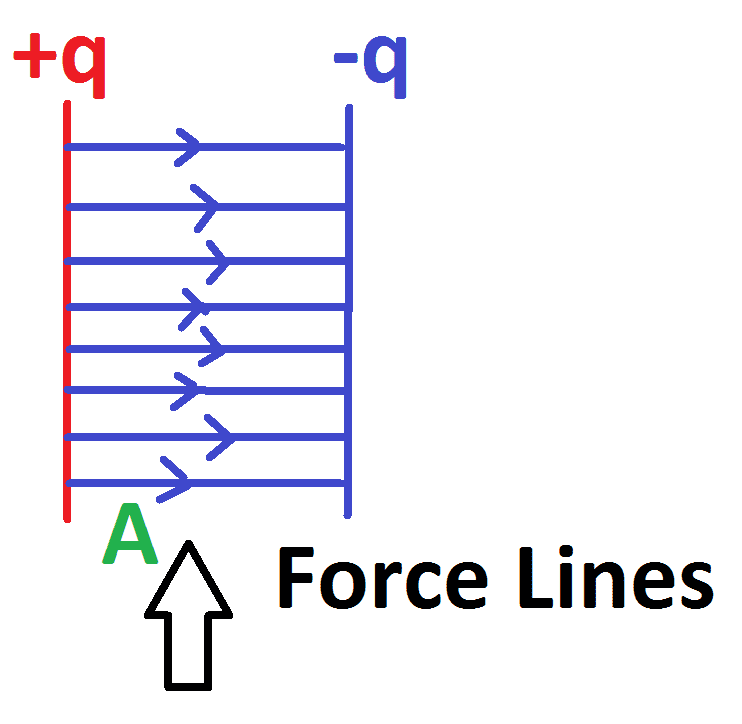Consider two charge sheets such as +q charge and –q charge and the area between the two charges is A. Electric field density can be defined as charge per unit area.